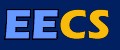# Randomized Rounding And Discrete Ham-Sandwich Theorems: Provably Good Algorithms for Routing and Packing Problems

### http://www.eecs.berkeley.edu/Pubs/TechRpts/1987/CSD-87-312.pdf

This thesis deals with the approximate solution of a class of zero-one integer programs arising in the design of integrated circuits, in operations research, and in some combinatorial problems. Our approach consists of first relaxing the integer program to a linear program, which can be solved by efficient algorithms. The linear program solution may assign fractional values to some of the variables, and these values are 'rounded' to obtain a provably good approximation to the original integer program.

We first consider the problem of global routing in gate-arrays. This problem has important applications in the design of integrated circuits, and can be formulated as a zero-one integer program. We introduce a technique we call randomized rounding for producing a provably good approximation to this integer program from the solution to its relaxation. In order to prove the quality of this approximation, we make use of some new bounds on the tail of the binomial distribution. We present the results of experiments conducted on industrial gate-arrays using our methods; these are encouraging and call for further work.

We then show that our randomized rounding technique can be applied to some problems in combinatorial optimization and operations research. We also describe the relation between the problems we study and a class of combinatorial results known as "discrete ham-sandwich theorems". This leads to the problem of rounding linear program solutions deterministically in polynomial time. We invoke an interesting "method of conditional probabilities" for this purpose. An extension of this method shows that it is possible to deterministically mimic the randomized algorithm in a certain precise sense. This leads us to the development of a deterministic polynomial time rounding algorithm that yields the same performance guarantees as the randomized method.

Advisor: Clark D. Thompson

BibTeX citation:

```@phdthesis{Raghavan:CSD-87-312,
Author = {Raghavan, Prabhakar},
Title = {Randomized Rounding And Discrete Ham-Sandwich Theorems: Provably Good Algorithms for Routing and Packing Problems},
School = {EECS Department, University of California, Berkeley},
Year = {1986},
Month = {Jul},
URL = {http://www.eecs.berkeley.edu/Pubs/TechRpts/1986/5986.html},
Number = {UCB/CSD-87-312}
}
```

EndNote citation:

```%0 Thesis
%A Raghavan, Prabhakar
%T Randomized Rounding And Discrete Ham-Sandwich Theorems: Provably Good Algorithms for Routing and Packing Problems
%I EECS Department, University of California, Berkeley
%D 1986
%@ UCB/CSD-87-312
%U http://www.eecs.berkeley.edu/Pubs/TechRpts/1986/5986.html
%F Raghavan:CSD-87-312
```